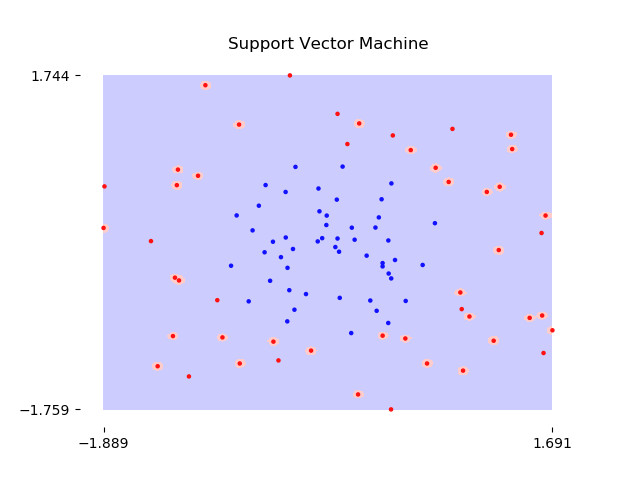# Learning Curve Examples

The difference between underfit (high bias), overfit (high variance), and appropriately fit models is shown below.

import pandas as pd
import numpy as np

data = pd.read_csv('learning-curve-examples/data.csv')
print(data.shape)
X = np.array(data[['x1', 'x2']])
y = np.array(data['y'])

(100, 3)

np.random.seed(42)


### Visualize Data

%matplotlib notebook

import matplotlib.pyplot as plt
from matplotlib.colors import ListedColormap

plt.figure()

cmap_bold  = ListedColormap(['#FF1111', '#1111FF'])
plt.scatter(X[:, 0],
X[:, 1],
s=20,
c=y,
cmap=cmap_bold,
zorder=3)

plt.grid(True, zorder=0)
for spine in plt.gca().spines.values():
spine.set_visible(False)
plt.tick_params(
axis='x',
bottom=False)
plt.tick_params(
axis='y',
left=False)

plt.title('The Data');

<IPython.core.display.Javascript object>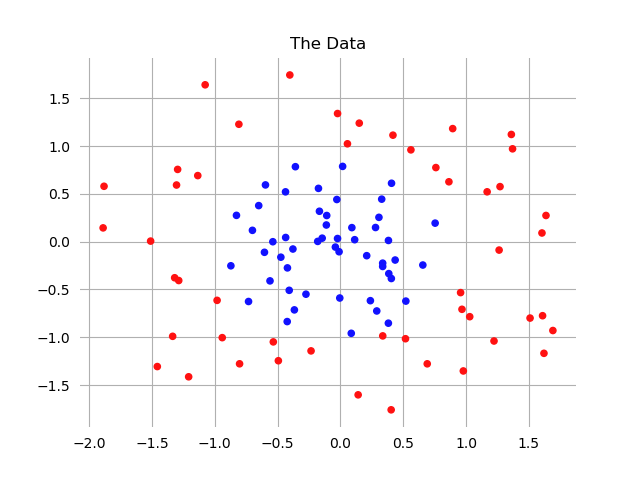### Initialize Four Machine Learning Models

from sklearn.model_selection import learning_curve
from sklearn.linear_model import LogisticRegression
from sklearn.svm import SVC

### Logistic Regression
logistic_regression = LogisticRegression()

### Decision Tree

### Support Vector Machine
support_vector_machine = SVC(kernel='rbf', gamma=1000)


### Define Utility Functions

Randomize Data

def randomize(X, Y):
permutation = np.random.permutation(Y.shape)
X2 = X[permutation,:]
Y2 = Y[permutation]

return X2, Y2

X_rand, y_rand = randomize(X, y)


Draw Learning Curves

def draw_learning_curves(estimator, title):
plt.figure()

train_sizes, train_scores, test_scores = (learning_curve(
estimator, X_rand, y_rand, cv=None, n_jobs=1,
train_sizes=range(5,85,5)))

train_scores_mean = np.mean(train_scores, axis=1)
train_scores_std = np.std(train_scores, axis=1)
test_scores_mean = np.mean(test_scores, axis=1)
test_scores_std = np.std(test_scores, axis=1)

plt.grid()

plt.title(title + " - Learning Curves")
plt.xlabel("Training examples")
plt.ylabel("Score")

plt.gca().set_ylim([0.35, 1.05])
for spine in plt.gca().spines.values():
spine.set_visible(False)
plt.tick_params(
axis='x',
bottom=False)
plt.tick_params(
axis='y',
left=False)

plt.fill_between(train_sizes, train_scores_mean - train_scores_std,
train_scores_mean + train_scores_std, alpha=0.1, color='g')
plt.fill_between(train_sizes, test_scores_mean - test_scores_std,
test_scores_mean + test_scores_std, alpha=0.1, color='y')
plt.plot(train_sizes, train_scores_mean, 'o-', color="g",
label="Training score")
plt.plot(train_sizes, test_scores_mean, 'o-', color="y",
label="Cross-validation score")

plt.legend(loc="best")

plt.show()


### Create Plots

for model, title in [(logistic_regression,    'Logistic Regression'),
(decision_tree,          'Decision Tree'),
(support_vector_machine, 'Support Vector Machine')]:
draw_learning_curves(model, title)

<IPython.core.display.Javascript object>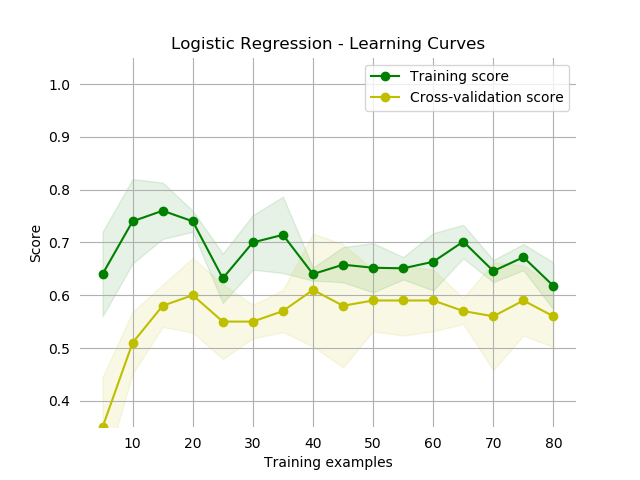<IPython.core.display.Javascript object>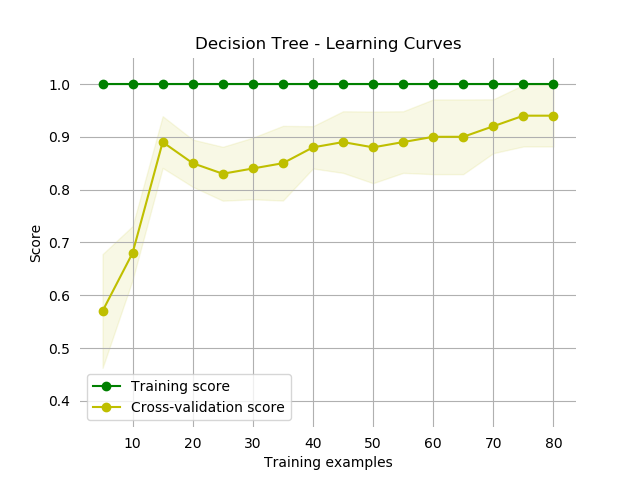<IPython.core.display.Javascript object>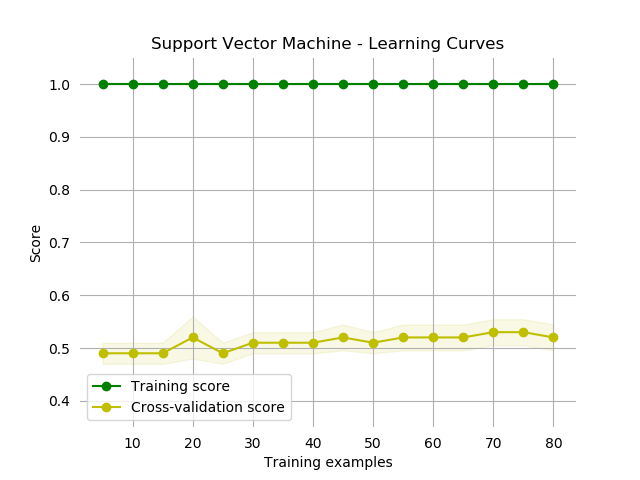The shapes shown above are very characteristic shapes. In particular:

• Logistic Regression: Underfits, both the training and cross-validation scores are low at less than 70%.

• Decision Tree: Appropriately Fit, the cross-validation score increases with more training examples, approaching the training score.

• Support Vector Machine: Overfits, the training score is high, but the cross-validation score never increases to approach it.

### Visualize the Decision Boundaries

The decision boundaries shown below demonstrate that these fit classifications are accurate.

from sklearn.model_selection import train_test_split

cmap_light = ListedColormap(['#FFCCCC', '#CCCCFF'])
cmap_bold = ListedColormap(['#FF1111', '#1111FF'])

mesh_step_size = .01
mark_symbol_size = 5

def plot_decision_boundaries(model, title):
plt.figure()

X_train, X_test, y_train, y_test = train_test_split(X_rand, y_rand, random_state=42)

model.fit(X_train, y_train)

x_min = X_rand[:,0].min()
x_max = X_rand[:,0].max()
y_min = X_rand[:,1].min()
y_max = X_rand[:,1].max()

# Plot Decision Boundaries
xx, yy = np.meshgrid(np.arange(x_min, x_max, mesh_step_size),
np.arange(y_min, y_max, mesh_step_size))
Z = model.predict(np.c_[xx.ravel(),
yy.ravel()])
Z = Z.reshape(xx.shape)
plt.pcolormesh(xx, yy, Z, cmap=cmap_light)

# Plot Points
plt.scatter(X_rand[:, 0],
X_rand[:, 1],
s=mark_symbol_size,
c=y_rand,
cmap=cmap_bold)
plt.title(title)

for spine in plt.gca().spines.values():
spine.set_visible(False)
plt.xticks([x_min,x_max])
plt.yticks([y_min,y_max]);

for model, title in [(logistic_regression,    'Logistic Regression'),
(decision_tree,          'Decision Tree'),
(support_vector_machine, 'Support Vector Machine')]:
plot_decision_boundaries(model, title)

<IPython.core.display.Javascript object>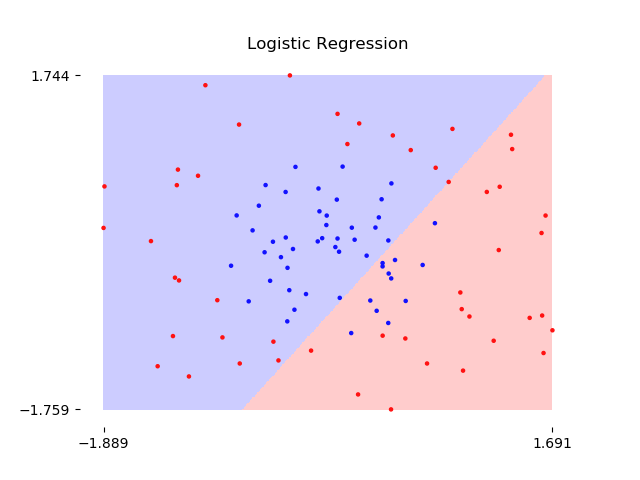<IPython.core.display.Javascript object>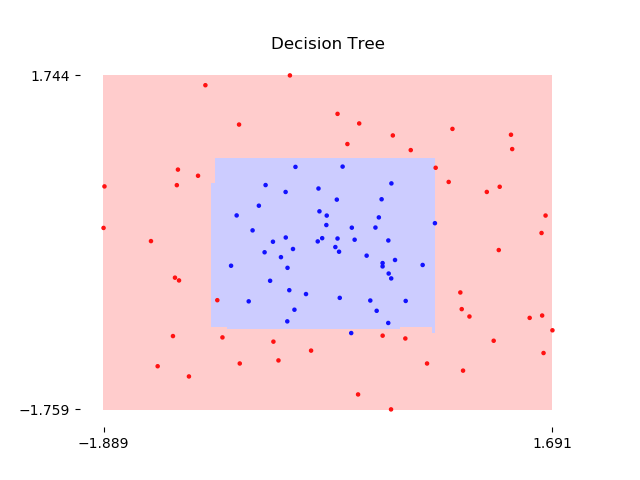<IPython.core.display.Javascript object>